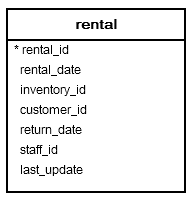# PostgreSQL Tutorial: AGE Function: Calculate Ages

September 19, 2023

Summary: in this tutorial, you will learn how to use the PostgreSQL `AGE()` function to calculate ages.

## Introduction to PostgreSQL age() function

We typically have to calculate ages in business applications e.g., ages of people, years of services of employees, etc. In PostgreSQL, you can use the `AGE()` function to achieve these tasks.

The following illustrates the syntax of the `AGE()` function:

``````AGE(timestamp,timestamp);
``````

The `AGE()` function accepts two `TIMESTAMP` values. It subtracts the second argument from the first one and returns an interval as a result.

See the following example:

``````SELECT AGE('2017-01-01','2011-06-24');
``````
``````          AGE
-----------------------
5 years 6 mons 7 days
(1 row)
``````

If you want to take the current date as the first argument, you can use the following form of the `AGE()` function:

``````AGE(timestamp);
``````

For example, if someone has a birth date`2000-01-01` and the current date is `2017-03-20`, his/her age will be:

``````SELECT current_date,
AGE(timestamp '2000-01-01');
``````
``````    date    |           AGE
------------+-------------------------
2017-03-20 | 17 years 2 mons 19 days
(1 row)
``````

## PostgreSQL age function example

See the following `rental` table in the sample database:Suppose you want to get the top 10 rentals that have the longest durations, you can use the `AGE()` function to calculate it as follows:

``````SELECT rental_id,
customer_id,
AGE(return_date,
rental_date) AS duration
FROM rental
WHERE return_date IS NOT NULL
ORDER BY  duration DESC
LIMIT 10;
``````

In this example, use the `AGE()` function to calculate the rental duration based on the values of the `rental_date` and `return_date` columns. The following shows the output:

`````` rental_id | customer_id |    duration
-----------+-------------+-----------------
2412 |         127 | 9 days 05:59:00
14678 |         383 | 9 days 05:59:00
13947 |         218 | 9 days 05:58:00
14468 |         224 | 9 days 05:58:00
7874 |          86 | 9 days 05:58:00
11629 |         299 | 9 days 05:58:00
5738 |         187 | 9 days 05:56:00
9938 |          63 | 9 days 05:56:00
12159 |         106 | 9 days 05:55:00
3873 |         394 | 9 days 05:55:00
(10 rows)
``````

In this tutorial, you have learned how to use the PostgreSQL `AGE()` function to calculate ages.